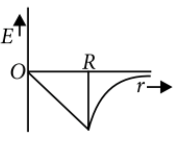Variation of g
Question

# Dependence of intensity of gravitational field (E) of earth with distance (r) from centre of earth is correctly represented by

Easy
Solution

## For a point inside the earth i.e. r < R$E=-\frac{GM}{{R}^{3}}r$where M and R be mass and radius of the earth respectively. At the centre, r = 0For a point outside the earth i.e. r > R, $E=-\frac{GM}{{r}^{2}}$  On the surface of the earth i.e. r = R$E=-\frac{GM}{{R}^{2}}$The variation of E with distance r from the centre is as shown in the figure.Get Instant Solutions